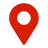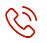【买车就上粒子汽车网，特卖更给力】北京400-6500-365

询问底价

5621397 客户通过询价购得心仪车辆
• 选择城市：
北京市
• 北京市
• 天津市
• 河北省
• 山西省
• 内蒙古自治区
• 辽宁省
• 吉林省
• 黑龙江省
• 上海市
• 江苏省
• 浙江省
• 安徽省
• 福建省
• 江西省
• 山东省
• 河南省
• 湖北省
• 湖南省
• 广东省
• 广西壮族自治区
• 海南省
• 重庆市
• 四川省
• 贵州省
• 云南省
• 西藏自治区
• 陕西省
• 甘肃省
• 青海省
• 宁夏回族自治区
• 新疆维吾尔自治区
北京
• A 安庆
• A 安阳
• A 鞍山
• A 安顺
• A 安康
• A 阿克苏地区
• B 北京
• B 保定
• B 包头
• B 保定涿州
• B 包头青山
• B 巴彦淖尔
• B 亳州
• B 滨州
• B 蚌埠
• B 百色
• B 本溪
• B 北京海淀
• B 保山
• B 毕节地区
• B 保山腾冲
• B 白银
• B 宝鸡
• B 巴音郭楞蒙古自治州
• B 博尔塔拉蒙古自治州
• C 沧州
• C 长治
• C 赤峰
• C 沧州黄骅
• C 沧州任丘
• C 承德
• C 沧州运河
• C 常州
• C 滁州
• C 池州
• C 长沙
• C 常德
• C 郴州
• C 潮州
• C 长春
• C 朝阳
• C 成都
• C 楚雄彝族自治州
• C 昌吉回族自治州
• D 大同
• D 东营
• D 德州
• D 东莞
• D 大连
• D 大庆
• D 丹东
• D 大连瓦房店
• D 德阳
• D 大理白族自治州
• D 德宏傣族景颇族自治州
• D 大理剑川
• D 达州
• D 定西
• E 鄂尔多斯
• E 鄂尔多斯达旗
• E 恩施土家族苗族自治州
• F 福州
• F 阜阳
• F 佛山
• F 佛山南海
• F 阜新
• F 抚顺
• G 赣州
• G 广州
• G 桂林
• G 广州海珠
• G 贵港
• G 贵阳
• G 广元
• G 固原
• H 邯郸
• H 衡水
• H 呼和浩特
• H 邯郸武安
• H 呼和浩特武川
• H 呼和浩特托县
• H 呼伦贝尔
• H 湖州
• H 杭州
• H 淮安
• H 合肥
• H 淮北
• H 淮南
• H 菏泽
• H 湖州长兴
• H 合肥蜀山
• H 黄石
• H 怀化
• H 衡阳
• H 黄冈
• H 惠州
• H 海口
• H 河源
• H 惠州惠阳
• H 河池
• H 哈尔滨
• H 黑河
• H 葫芦岛
• H 红河哈尼族彝族自治州
• H 汉中
• H 哈密地区
• J 晋中
• J 晋城
• J 济南
• J 嘉兴
• J 金华
• J 济宁
• J 景德镇
• J 九江
• J 济宁邹城
• J 济南章丘
• J 济宁曲阜
• J 嘉兴海宁
• J 吉安
• J 金华东阳
• J 荆州
• J 焦作
• J 荆门
• J 江门
• J 揭阳
• J 江门新会
• J 佳木斯
• J 锦州
• J 吉林
• K 开封
• K 昆明
• K 克拉玛依
• K 喀什地区
• L 廊坊
• L 临汾
• L 吕梁
• L 廊坊霸州
• L 临沂
• L 龙岩
• L 六安
• L 连云港
• L 聊城
• L 丽水
• L 莱芜
• L 洛阳
• L 娄底
• L 漯河
• L 柳州
• L 来宾
• L 辽阳
• L 丽江
• L 六盘水
• L 拉萨
• L 乐山
• L 凉山彝族自治州
• L 泸州
• L 临沧
• L 兰州
• M 马鞍山
• M 梅州
• M 茂名
• M 牡丹江
• M 绵阳
• M 眉山
• N 南通
• N 宁波
• N 南京
• N 南昌
• N 宁波余姚
• N 南通如东
• N 宁波象山
• N 南平
• N 南通海安
• N 宁德
• N 南阳
• N 南阳宛城
• N 南宁
• N 内江
• N 南充
• P 莆田
• P 萍乡
• P 平顶山
• P 濮阳
• P 盘锦
• P 攀枝花
• P 普洱
• Q 秦皇岛
• Q 青岛
• Q 泉州
• Q 衢州
• Q 青岛平度
• Q 青岛胶州
• Q 青岛莱西
• Q 青岛黄岛
• Q 泉州石狮
• Q 潜江
• Q 清远
• Q 琼海
• Q 钦州
• Q 齐齐哈尔
• Q 曲靖
• Q 黔东南苗族侗族自治州
• Q 黔南布依族苗族自治州
• Q 黔西南布依族苗族自治州
• R 日照
• R 日喀则地区
• S 上海
• S 石家庄
• S 石家庄辛集
• S 朔州
• S 绍兴
• S 苏州
• S 宿迁
• S 宿州
• S 上饶
• S 苏州张家港
• S 苏州吴江
• S 宿迁沭阳
• S 苏州昆山
• S 上海浦西
• S 上海闵行
• S 上海浦东
• S 上海静安
• S 十堰
• S 三门峡
• S 商丘
• S 商丘永城
• S 邵阳
• S 随州
• S 深圳
• S 汕尾
• S 韶关
• S 汕头
• S 三亚
• S 深圳宝安
• S 沈阳
• S 松原
• S 首都北京
• S 遂宁
• S 商洛
• S 石嘴山
• T 天津
• T 唐山
• T 太原
• T 唐山迁安
• T 通辽
• T 唐山丰润
• T 通辽科尔沁
• T 台州
• T 泰州
• T 泰安
• T 天津滨海
• T 泰州泰兴
• T 铜陵
• T 铜陵枞阳
• T 铁岭
• T 通化
• T 铜仁地区
• T 铜川
• T 天水
• W 乌海
• W 乌兰察布
• W 无锡
• W 温州
• W 潍坊
• W 芜湖
• W 威海
• W 潍坊诸城
• W 无锡宜兴
• W 温州乐清
• W 无锡江阴
• W 温州苍南
• W 潍坊青州
• W 武汉
• W 文山壮族苗族自治州
• W 渭南
• W 乌鲁木齐
• W 吴忠
• W 武威
• X 邢台
• X 锡林郭勒盟
• X 忻州
• X 兴安盟
• X 厦门
• X 徐州
• X 新余
• X 宣城
• X 徐州邳州
• X 襄阳
• X 新乡
• X 信阳
• X 湘潭
• X 湘西土家族苗族自治州
• X 许昌
• X 咸宁
• X 孝感
• X 新乡长垣
• X 西双版纳傣族自治州
• X 西安
• X 咸阳
• X 西宁市
• X 新疆
• Y 运城
• Y 阳泉
• Y 盐城
• Y 扬州
• Y 烟台
• Y 鹰潭
• Y 宜春
• Y 烟台龙口
• Y 烟台莱州
• Y 烟台招远
• Y 岳阳
• Y 永州
• Y 宜昌
• Y 益阳
• Y 玉林
• Y 阳江
• Y 延边朝鲜族自治州
• Y 营口
• Y 雅安
• Y 玉溪
• Y 宜宾
• Y 银川
• Y 延安
• Y 榆林
• Y 伊犁哈萨克自治州
• Z 重庆
• Z 张家口
• Z 镇江
• Z 漳州
• Z 淄博
• Z 舟山
• Z 枣庄
• Z 枣庄滕州
• Z 镇江丹阳
• Z 郑州
• Z 驻马店
• Z 株洲
• Z 张家界
• Z 郑州中牟
• Z 周口
• Z 中山
• Z 珠海
• Z 肇庆
• Z 湛江
• Z 遵义
• Z 遵义仁怀
• Z 自贡
• Z 重庆万州
• Z 资阳
• Z 昭通
• Z 中卫
• Z 张掖
• 意向车型：
选择品牌
• A 奥迪
• A 阿尔法·罗密欧
• B 别克
• B 北京汽车
• B 北京现代
• B 北汽新能源
• B 比亚迪
• B 比亚迪新能源
• B 宝马
• B 北汽福田
• B 奔驰
• B 宝骏
• B 宝沃
• B 北汽威旺
• B 北汽幻速
• B 保时捷
• B 比速
• B 宾利
• B 宝马MINI
• B 宝骏新能源
• B 奔驰AMG
• B 比亚迪e购
• C 长安福特
• C 纯电动能源
• C 长安汽车
• C 长安商用
• C 长安铃木
• C 长安马自达
• C 昌河汽车
• C 长城汽车
• C 长丰汽车
• C 传祺新能源
• C 长安新能源
• C 昌河铃木
• C 长安微面
• C 长安轻型车
• D 东风日产
• D 东风本田
• D DS
• D 东风风神
• D 东风风行
• D 东风标致
• D 东风悦达起亚
• D 东风雪铁龙
• D 东风小康
• D 东风风光
• D 东风风度
• D 东南三菱
• D 东风御风
• D 东南汽车
• D 大乘汽车
• F 福特全顺
• F 福建奔驰
• F 福迪汽车
• F 福汽启腾
• F 法拉利
• F 房车
• G 广汽新能源
• G 广汽传祺
• G 广汽本田
• G 广汽丰田
• G 广汽三菱
• G 观致
• G 广汽菲克JEEP
• G 广汽菲亚特
• G 广汽吉奥
• G GMC
• H 华晨宝马
• H 哈弗
• H 汉腾
• H 海马汽车
• H 华泰汽车
• H 华晨金杯
• H 黄海汽车
• H 海马新能源
• H 哈弗蓝标
• H 哈弗红标
• H 华晨雷诺
• J 进口福特
• J 吉利汽车
• J 吉普
• J 江铃汽车
• J 江淮汽车
• J 江淮新能源
• J 捷豹
• J 吉利新能源
• J 进口大众
• J 进口三菱
• J 进口起亚
• J 进口丰田
• J 捷途
• J 吉利L网
• J 进口现代
• J 九龙汽车
• J 江西五十铃
• J 江铃轻汽
• J 江铃福特
• J 进口日产
• J 吉利G网
• J 君马汽车
• J 进口铃木
• J 江淮卡车
• J 吉利汽车L网
• J 进口奥迪
• J 江铃新能源
• K 凯迪拉克
• K 克莱斯勒
• K 酷威
• K 凯翼汽车
• K 开瑞汽车
• L 林肯
• L 陆风
• L 路虎
• L 雷诺
• L 力帆
• L 雷克萨斯
• L 猎豹
• L 兰博基尼
• L 领克
• M 玛莎拉蒂
• M 名爵
• N 纳智捷
• N 南京依维柯
• N 哪吒汽车
• O 讴歌
• O 欧拉ORA
• P 平行进口车
• P 皮卡
• Q 奇瑞新能源
• Q 奇瑞
• Q 启辰
• Q 前途
• Q 庆铃汽车
• R 荣威
• R 荣威新能源
• S 上汽大众
• S 斯柯达
• S 上汽大通
• S 双龙汽车
• S 斯巴鲁
• S 斯威汽车
• S 四川现代
• S SMART
• T 腾势
• T 天津一汽
• T 特斯拉
• W 五菱
• W 沃尔沃
• W WEY
• W 威马汽车
• W 蔚来
• X 雪佛兰
• X 厦门金龙
• X 小鹏汽车
• X 新特
• X 星途
• Y 一汽大众
• Y 英菲尼迪
• Y 一汽红旗
• Y 一汽奔腾
• Y 一汽森雅
• Y 一汽丰田
• Y 一汽马自达
• Y 云度新能源
• Y 野马汽车
• Y 英致
• Y 御捷新能源
• Y 一汽佳宝
• Z 知豆
• Z 众泰新能源
• Z 众泰S系列
• Z 众泰T系列
• Z 郑州日产
• Z 中华
• Z 郑州海马汽车
• Z 中兴
• Z 众泰大迈
• Z 众泰Z系列
• Z 中规进口车
选择车型
• 您的姓名：
• 手机号码：